### IMO Shortlist 2017 problem C5

Kvaliteta:
Avg: 0.0
Težina:
Avg: 8.0

A hunter and an invisible rabbit play a game in the Eulidean plane. The hunter's starting point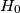$H_0$ coincides with the rabbit's starting point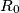$R_0$. In the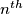$n^{th}$ th round of the game (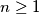$n \geq 1$), the following happens.

(1) First the invisible rabbit moves secretly and unobserved from its current point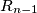$R_{n-1}$ to some new point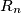$R_n$ with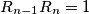$R_{n-1}R_n = 1$.

(2) The hunter has a tracking device (e.g. dog) that returns an approximate position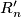$R'_n$ of the rabbit, so that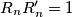$R_nR'_n = 1$.

(3) The hunter then visibly moves from point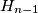$H_{n-1}$ to a new point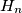$H_n$ with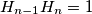$H_{n-1}H_n = 1$.

Is there a strategy for the hunter that guarantees that after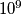$10^9$ such rounds the distance between the hunter and the rabbit is below 100?

Source: https://www.imo-official.org/problems/IMO2017SL.pdf